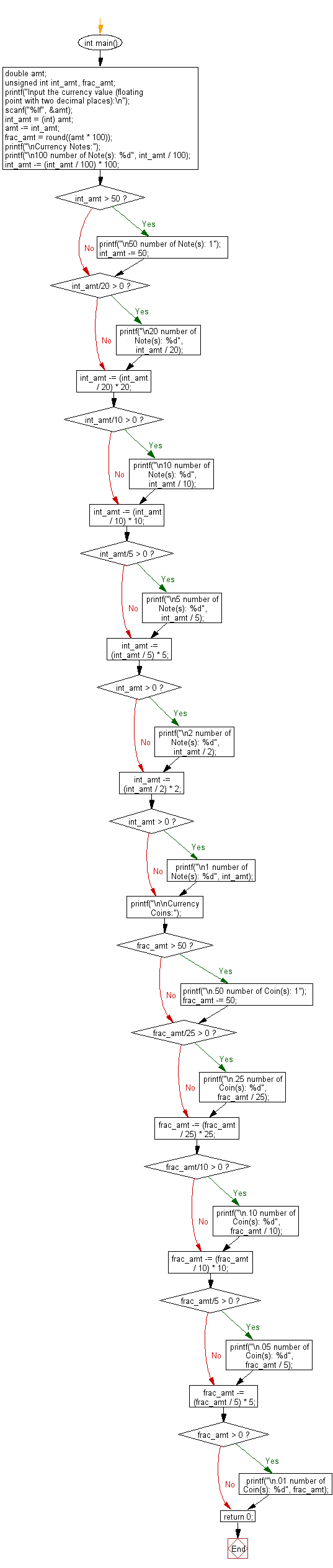﻿ C Program: Convert a given amount to notes and coins

# C Exercises: Convert a given amount to possible number of notes and coins

## C Basic Declarations and Expressions: Exercise-100 with Solution

Write a C program to convert a currency value (floating point with two decimal places) to the number of coins and notes.

Possible Notes: 100, 50, 20, 10, 5, 2, 1
Possible Coins: 0.50, 0.25, 0.10, 0.05 and 0.01

Sample Solution:

C Code:

``````#include <stdio.h>
#include <math.h>

int main() {
double amt;

unsigned int int_amt, frac_amt;

// Prompt the user to input the currency value
printf("Input the currency value (floating point with two decimal places):\n");

scanf("%lf", &amt);

// Separate the integer part and fractional part
int_amt = (int) amt;
amt -= int_amt;
frac_amt = round((amt * 100));

// Display currency notes
printf("\nCurrency Notes:");

// Calculate and display 100 rupee notes
printf("\n100 number of Note(s): %d", int_amt / 100);

// Update the integer amount after deducting 100 rupee notes
int_amt -= (int_amt / 100) * 100;

// Check and display 50 rupee note
if (int_amt > 50) {
printf("\n50 number of Note(s): 1");
int_amt -= 50;
}

// Check and display 20 rupee notes
if (int_amt/20 > 0)
printf("\n20 number of Note(s): %d", int_amt / 20);

// Update the integer amount after deducting 20 rupee notes
int_amt -= (int_amt / 20) * 20;

// Check and display 10 rupee notes
if (int_amt/10 > 0)
printf("\n10 number of Note(s): %d", int_amt / 10);

// Update the integer amount after deducting 10 rupee notes
int_amt -= (int_amt / 10) * 10;

// Check and display 5 rupee notes
if (int_amt/5 > 0)
printf("\n5 number of Note(s): %d", int_amt / 5);

// Update the integer amount after deducting 5 rupee notes
int_amt -= (int_amt / 5) * 5;

// Check and display 2 rupee notes
if (int_amt > 0)
printf("\n2 number of Note(s): %d", int_amt / 2);

// Update the integer amount after deducting 2 rupee notes
int_amt -= (int_amt / 2) * 2;

// Check and display 1 rupee notes
if (int_amt > 0)
printf("\n1 number of Note(s): %d", int_amt);

// Display currency coins
printf("\n\nCurrency Coins:");

// Check and display 50 paise coins
if (frac_amt > 50) {
printf("\n.50 number of Coin(s): 1");
frac_amt -= 50;
}

// Check and display 25 paise coins
if (frac_amt/25 > 0)
printf("\n.25 number of Coin(s): %d", frac_amt / 25);

// Update the fractional amount after deducting 25 paise coins
frac_amt -= (frac_amt / 25) * 25;

// Check and display 10 paise coins
if (frac_amt/10 > 0)
printf("\n.10 number of Coin(s): %d", frac_amt / 10);

// Update the fractional amount after deducting 10 paise coins
frac_amt -= (frac_amt / 10) * 10;

// Check and display 5 paise coins
if (frac_amt/5 > 0)
printf("\n.05 number of Coin(s): %d", frac_amt / 5);

// Update the fractional amount after deducting 5 paise coins
frac_amt -= (frac_amt / 5) * 5;

// Check and display 1 paise coins
if (frac_amt > 0)
printf("\n.01 number of Coin(s): %d", frac_amt);

return 0;
}
``````

Sample Output:

```Input the currency value (floating point with two decimal places):
10387.75

Currency Notes:
100 number of Note(s): 103
50 number of Note(s): 1
20 number of Note(s): 1
10 number of Note(s): 1
5 number of Note(s): 1
2 number of Note(s): 1

Currency Coins:
.50 number of Coin(s): 1
.25 number of Coin(s): 1
```

Flowchart:C programming Code Editor:

What is the difficulty level of this exercise?

Test your Programming skills with w3resource's quiz.

﻿